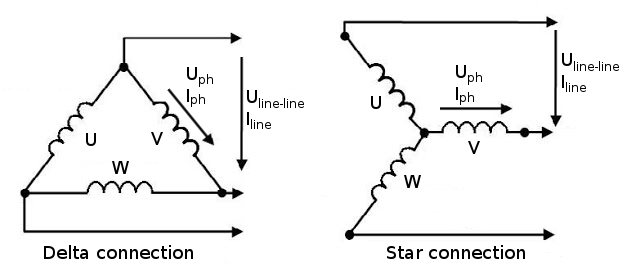# Delta Star connection

Two different possibilities to connect the three phases

The delta (Δ) connection is sometimes also called triangle, π (spelled out as 'pi'), or mesh connection.

The star (Y) connection is sometimes also spelled out as 'wye' or called as T connection. With the star connection, the neutral point is physically accessible, refer to Fig. 1.Fig. 1 Schemes for delta and star connection of machine windings.

When considering the phase voltages $U_{ph}$ and phase currents $I_{ph}$ of the three machine windings $U$, $V$, and $W$ as shown in Fig. 1, no information is necessary about whether your machine windings are delta or star connected.

The choice between delta and star connection of your machine windings is often dependent on the voltage and current ratings of your supply. In the table below, you can find relevant equations to determine the line quantities ($U_{line-line}$, $I_{line}$) of your supply from the phase quantities depending on the connection type.

 Connection type Delta (Δ) Star (Y) $U_{line-line}$ $U_{ph}$ $\sqrt{3}U_{ph}$ $I_{line}$ $\sqrt{3}I_{ph}$ $I_{ph}$